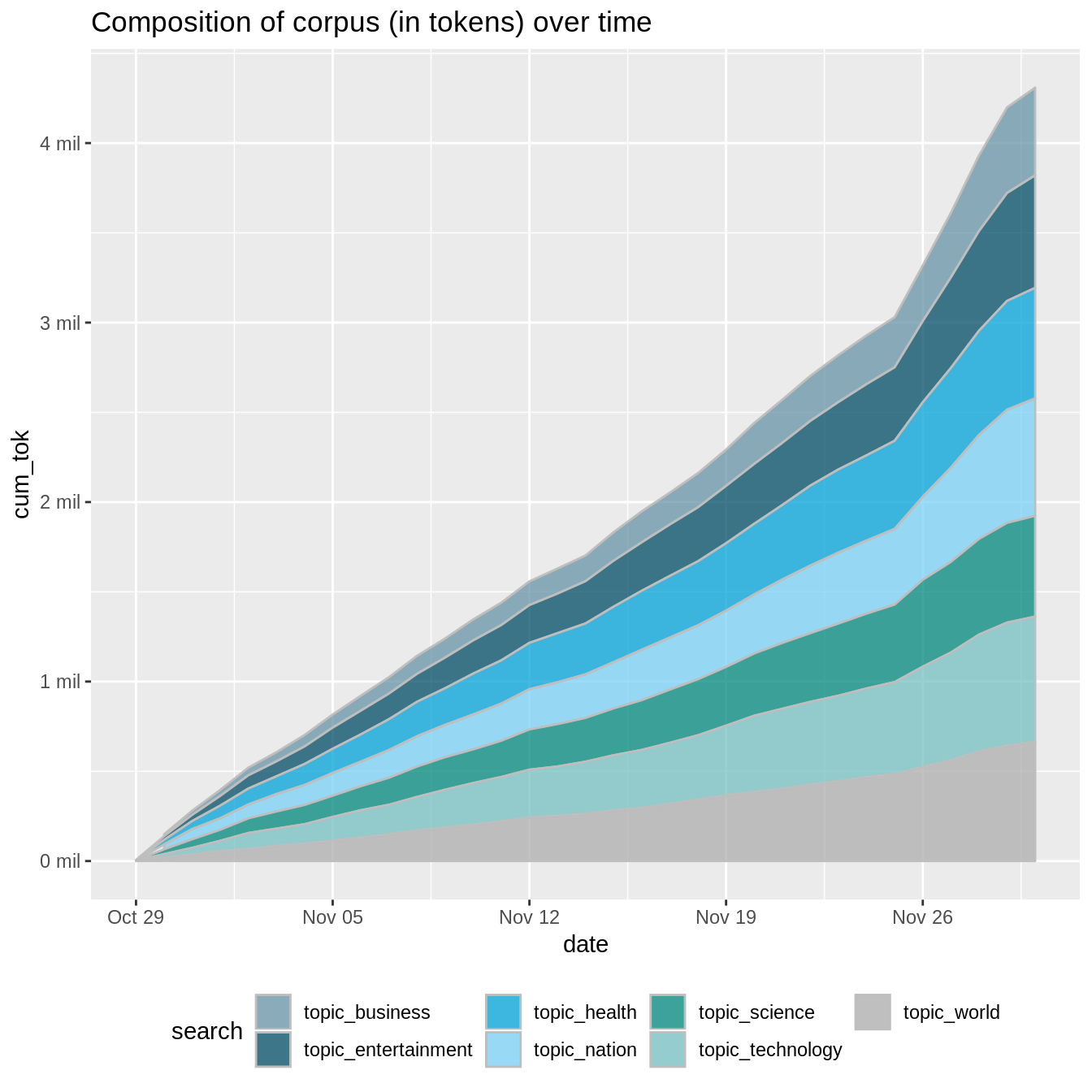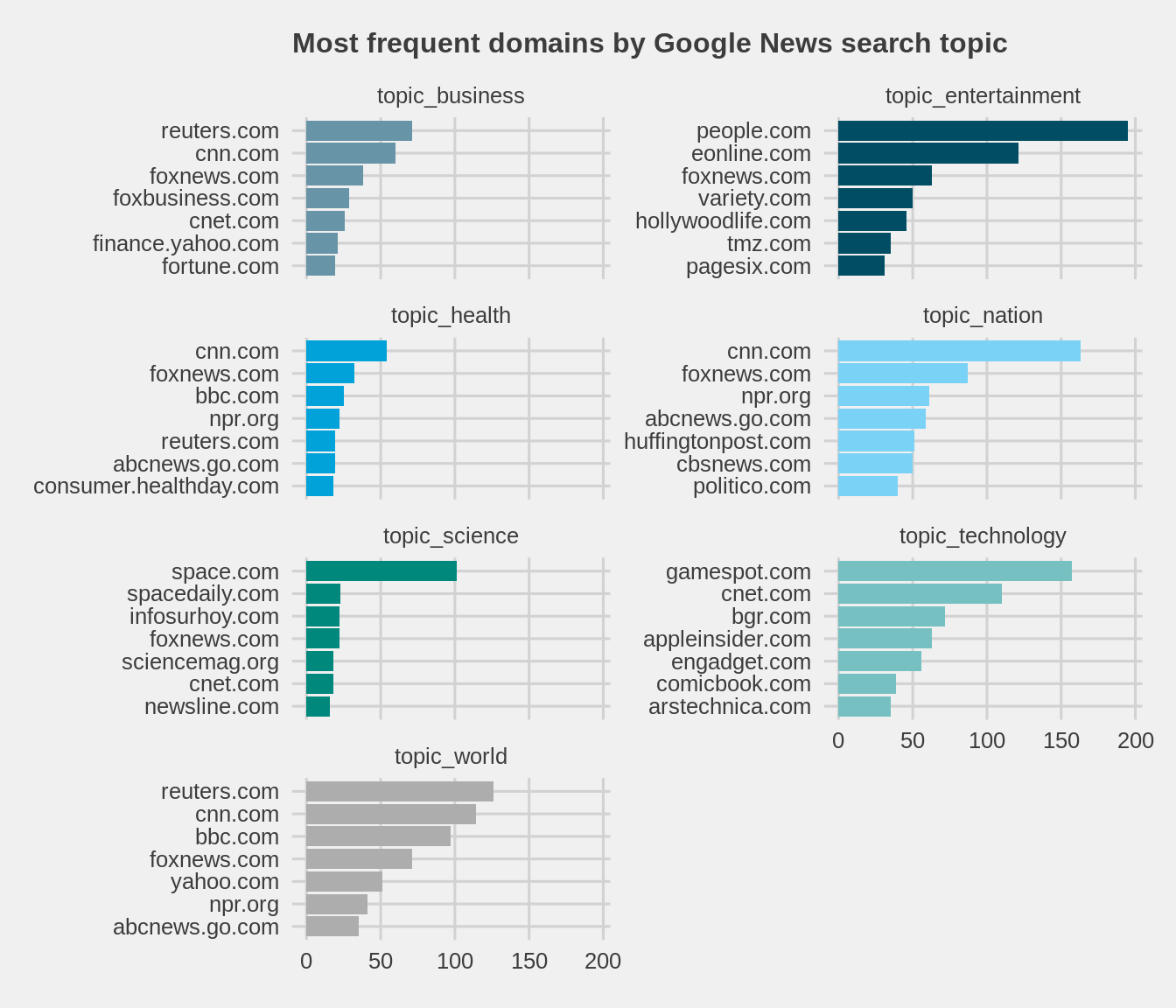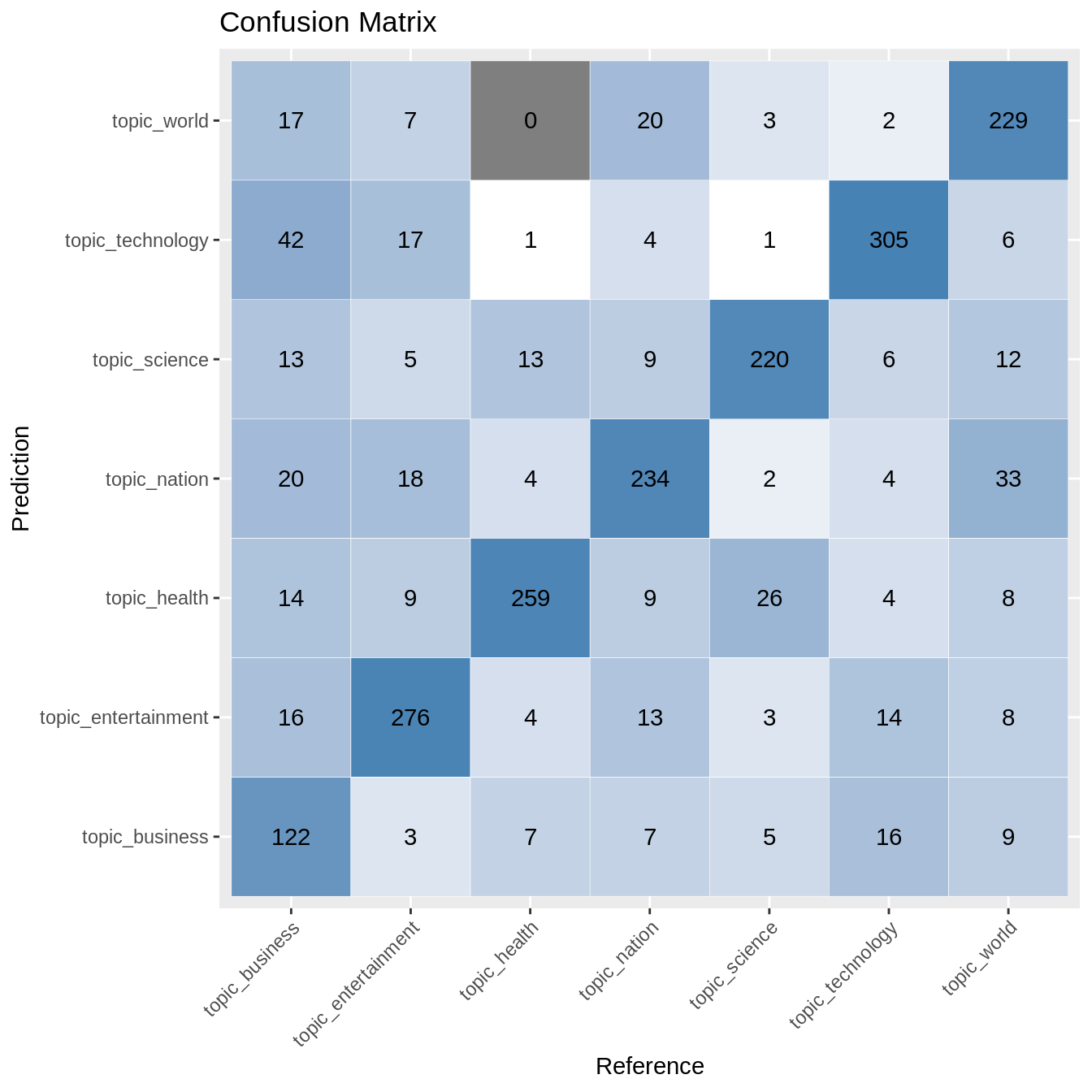# genre, text classification & naive bayes

In this short post, we outline a Naive Bayes (NB) approach to genre-based text classification. First, we introduce & describe a corpus derived from Google News’ RSS feed, which includes source and genre information. We then train, test & evaluate the efficacy of an NB classifier applied to online news genres, with some fairly nice results. Here, we focus on the nuts/bolts of an R-based workflow, and leave discussion of theory & Bayesian assumptions for another day.

library(e1071)
library(caret)
library(tidyverse)
library(knitr)
#library(quicknews)#devtools::install_github("jaytimm/quicknews")

## Building a historical, genre-based corpus

For demonstration purposes, I have built a fairly small corpus comprised of national news articles from Google News’ RSS feed. The corpus (as TIF) was built using my quicknews package, and assembled over the course of roughly one month (10/29/18 ~ 11/30/18). Article text & metadata were scraped/collected three times a day using the Windows task scheduler app. The R script used for corpus assembly (which should scale quite nicely to different/novel search types) is available here.

setwd(local1)
mutate(length = lengths(gregexpr("\\W+", text)) + 1)

Metadata include the genre (as defined by Google News) & domain name of article source.

colnames(qnews_tif)
##   "doc_id"  "link"    "lang"    "country" "search"  "date"    "source"
##   "title"   "text"    "length"

The table below summarizes the composition of our corpus in terms of genre. So, in roughly a month, we have assembled a ~6.9K text corpus comprised of ~4.3 million words. And fairly balanced as well from a genre perspective.

qnews_tif %>%
group_by(search) %>%
summarize(tokens = sum(length),
texts = length(unique(doc_id))) %>%
knitr::kable()
search tokens texts
topic_entertainment 627014 1119
topic_health 615731 961
topic_nation 654143 989
topic_science 561797 869
topic_technology 697857 1170
topic_world 663889 1019
Total 4309193 6941

The plot below illustrates the growth of our corpus (by genre) over time.

qnews_tif  %>%
group_by(date, search) %>%
summarize(tokens = sum(length)) %>%
group_by(search) %>%
mutate(cum_tok = cumsum(tokens))%>%
filter(tokens > 360) %>%

ggplot(aes(x=date, y=cum_tok, fill = search)) +
geom_area(alpha = 0.75, color = 'gray') +
ggthemes::scale_fill_economist()+
theme(legend.position = "bottom")+
scale_y_continuous(labels = function(x) paste0(format(x/1000000), ' mil')) +
labs(title = "Composition of corpus (in tokens) over time")Lastly, and largely for good measure, we take a quick look at corpus composition in terms of article sources. The plot below summarizes the top content generators within each genre as measured by article counts.

qnews_tif %>%
group_by(search, source) %>%
summarize(count = n()) %>%
arrange(search,(count))%>%
top_n(n=7,wt=jitter(count))%>%
ungroup()%>%
#Hack1 to sort order within facet
mutate(order = row_number(),
source=factor(paste(order,source,sep="_"),
levels = paste(order, source, sep = "_")))%>%
ggplot(aes(x=source,
y=count,
fill=search)) +
geom_col(show.legend = FALSE) +
facet_wrap(~search, scales = "free_y", ncol = 2) +
#Hack2 to modify labels
scale_x_discrete(labels = function(x) gsub("^.*_", "", x))+
ggthemes::theme_fivethirtyeight()+
ggthemes::scale_fill_economist() +
theme(plot.title = element_text(size=12))+
coord_flip()+
labs(title="Most frequent domains by Google News search topic")## Building a Naive Bayes classifier

To build a Naive Bayes classifier via caret, we first need to transform the tif corpus to a document-term matrix (DTM). Here, we work within the text2vec framework (see vignette here). Document vectors are L1-normalized and transformed via TF-IDF. Note that there are any number of other ways to transform a tif corpus to a DTM. Despite some idiosyncratic syntax, text2vec is super-quick & super-flexible, and likely a bit overkill here.

t2v_corp <-  text2vec::itoken(qnews_tif$text, preprocessor = tolower, tokenizer = text2vec::word_tokenizer, ids = qnews_tif$doc_id)

vocab <- text2vec::create_vocabulary(t2v_corp,
stopwords = tm::stopwords()) %>%
text2vec::prune_vocabulary(doc_proportion_min = .01,
doc_proportion_max = .4) %>%
arrange(term)

dtm <- text2vec::create_dtm(t2v_corp,
text2vec::vocab_vectorizer(vocab))

model_tfidf = text2vec::TfIdf$new() dtm <- model_tfidf$fit_transform(dtm)

Using the caret package, then, we divide the above matrix into a training set (as 70% of full data set) and a test set (as 30%). The createDataPartition function conveniently creates two equally proportioned samples.

set.seed(99)
trainIndex <- caret::createDataPartition(qnews_tif$search, p=0.7)$Resample1
train_data <- dtm[trainIndex, ]
test_data <- dtm[-trainIndex, ] #Demo distributions.

With the naiveBayes function from the e1071 package, we build our Naive Bayes classifier based on the training portion of the document-term matrix.

qnews_tif$search <- as.factor(qnews_tif$search)

classifier <- e1071::naiveBayes(as.matrix(train_data),
qnews_tif[trainIndex, ]$search, laplace = 0.5)  Then we implement the classifier on the test portion of the document-term matrix. test_predicted <- predict(classifier, as.matrix(test_data)) Output contains a vector of genre predictions for each text in the test data set. eg: head(test_predicted) ##  topic_nation topic_world topic_health topic_health topic_science ##  topic_science ## 7 Levels: topic_business topic_entertainment ... topic_world ## Model assessment & confusion matrix So, to get a sense of classifier efficacy in identifying the genre of a given article posted on Google News, we calculate a cross-tab of observed & predicted genres via the confusionMatrix function from caret. cfm <- caret::confusionMatrix(data = test_predicted, qnews_tif[-trainIndex, ]$search)

Overall fitness statistics of our model can be accessed via the overall element from the list of outputs generated by the confusionMatrix function. So, classifier accuracy is quite good at ~ 80%. I am sure this is not at gold standard levels; however, seemingly alright for a simple token-based approach to text classification.

cfm$overall ## Accuracy Kappa AccuracyLower AccuracyUpper AccuracyNull ## 7.912458e-01 7.556156e-01 7.731304e-01 8.085361e-01 1.688312e-01 ## AccuracyPValue McnemarPValue ## 0.000000e+00 9.853986e-08 Lastly, we visualize the confusion matrix based on the table element of output as a tile plot below. Tiles in the most prominent shade of blue reflect correct classifications. ggplot(data = as.data.frame(cfm$table) ,
aes(x = Reference, y = Prediction)) +
geom_tile(aes(fill = log(Freq)),
colour = "white") +
high = "steelblue") +
geom_text(aes(x = Reference,
y = Prediction,
label = Freq)) +
theme(legend.position = "none",
axis.text.x=element_text(angle=45,
hjust=1)) +
labs(title="Confusion Matrix")Output from the confusionMatrix function also includes a host of model diagnostics. The table below summarizes some of the more common diagnostics by genre, including sensitivity (1 - proportion of false negatives), specificity (1 - proportion of false positives), and the average of the two, balanced accuracy.

cfm\$byClass %>% data.frame() %>%
select (Sensitivity, Specificity, Balanced.Accuracy) %>%
rownames_to_column(var = 'topic') %>%
mutate(topic = gsub('Class: ','', topic)) %>%
mutate_if(is.numeric, round, 2) %>%
knitr::kable() 
topic Sensitivity Specificity Balanced.Accuracy